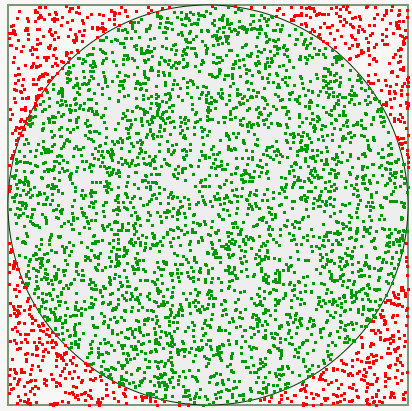# Monte Carlo Experiment

· Performance Zone · Tutorial
Save
3.67K Views

Monte Carlo simulations are a powerful way to solve a bunch of problems using random sampling. These include, and are not limited to, estimating computations that are costly in nature.

Here’s a fun way of computing value of π. Consider a circle of radius r centered at (0,0). This could be bound by a square with corners at (-r, -r), and (r, r). The area of the circle is πr² while the area of the binding square is 4r². If you were to randomly mark n points within the square, the probability p that it would be within the circle will be area of circle / area of square => π/4.

In a Monte Carlo experiment, you would randomly mark n points, and measure the probability p by counting the number of points within the circle. You have,

p/n = πr²/4r²

or

π = 4p/n.